Project

# A Polynomial Surface Resembling the Future Sketches Logo

Creative Commons

Lingdong Huang

##### Groups

(Or, How to Find an Equation for Every Picture)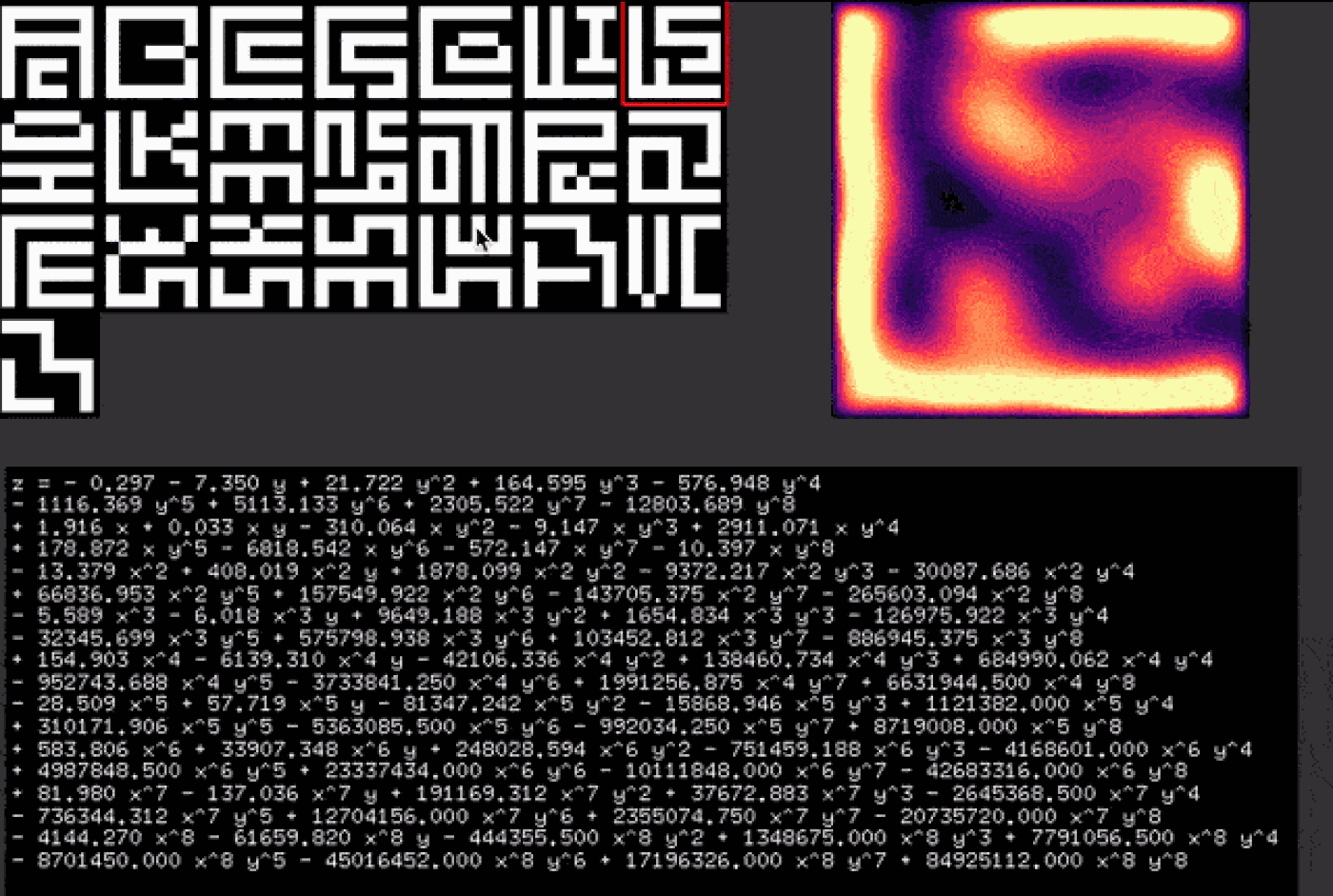Creative Commons

When developing examples for my lambda-2D programming language, prof. Zach gave me an interesting challenge: can you draw a program in the shape of the Future Sketches logo, that outputs the Future Sketches logo?

Half of the challenge was of course to arrange the symbols in the program to resemble the logo, but the other half struck me as an interesting problem on its own: How to describe a logo with a program?

It might sound obvious; One could of course iterate through every pixel of the logo and state whether it is white or black -- but that would be boring. What would be some ways to describe an image, without explicitly describing the image?

For the self-drawing lambda-2D program, I ended up making it a neat little demo for run-length encoding. But the search for more interesting and implicit methods to describe an image goes on.

The idea of having an equation of a 3D surface, that, when thresholded along Z-axis, produces a binary image resembling the logo came into mind. I vaguely recall that there're those things called Fourier synthesis and discrete cosine transform, which could be useful for this sort of thing. But then a much more evil and hideous thought surfaced: Could I simply fit a polynomial to a bunch of points that I know are on the surface?

We've all used the least squares method to fit polynomials to a bunch of 2D points, surely it'll work for 3D points as well? As long as we're happy to go into higher and higher order polynomials, we could express images of higher and higher compexity. The sheer brute-force mentality of this idea excites me.

A quick surf on the Internet confirms that the least squares method is indeed capable of doing that, albeit it seems prone to numerical accuracy issues. This particular StackOverflow answer  helped me figure out the functions I need to call in numpy to make it happen.

It is rather easy to understand: say you have points (x0,y0,z0), (x1,y1,z1), and (x2,y2,z2) and want to fit a 2nd order polynomial to them. So eventually you want an equation that looks like: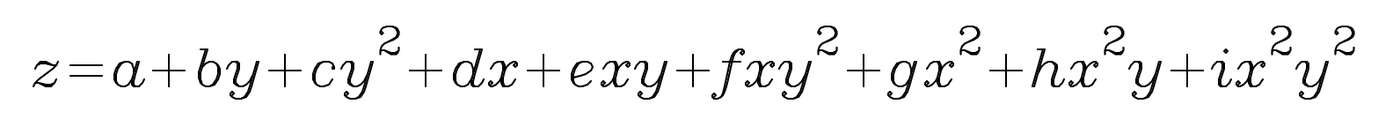Creative Commons

You have (2+1)^2=9 coefficients to figure out. Therefore, you pose it as a linear algebra problem, in which you want to find matrix M containing all the coefficients such that G * M = Z, where G is: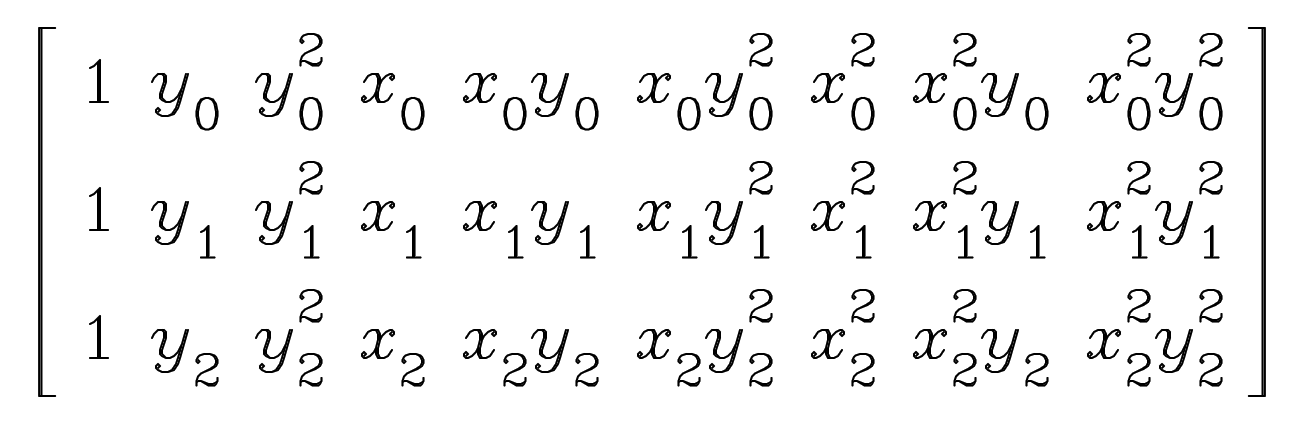Creative Commons

and M is [a,b,c,d,e,f,g,h,i]^T, and Z is [z0,z1,z2]^T. (Please excuse the lack of proper formatting due to limitations of the Media Lab website editor).

The system would be over-determined when you have many points, that's why we use the least-squares method, which is a way of saying "If we can't make every point perfectly happy, we make them mostly quite happy overall".

I've previously written a linear algebra library from scratch in JavaScript, in which I implemented a function for least squares approximation. However my linear algebra skills has since deteriorated, so please forgive me (and let me know) if I made any errors in the above simplified explanation!

Now it's time to prepare the points to feed into our solver! Given a binary image of dimension N x N, we can genearte N^2 points, whose x and y coordinates are their position (column, row) in the image, and z can be either -1 (black) or 1 (white). Though z can be in fact any two values for black and white, it seems that normalizing around 0 gives best numerical accuracy. (Yes, in case you don't know already, due to the way computers store floating point numbers, math is best done when operands are close to zero.) For the same reason, the x and y coordinates are normalized to (-0.5, 0.5) range.

Next we need to determine the order of the polynomial needed. Here's my intuition: the graph of y=x has no valley or hills. The graph of y=x^2 has one valley. The graph of y=x^3+x^2 has one valley and one hill. The graph of y=x^4-x^3-x^2 has two valleys and one hill. And so on. Therefore, if your image has N valleys or hills on either X or Y axis, you probably need a polynomial of order somewhere around N+1.

Playing around with the numbers confirmed my hypothesis: with the 7x7 Future Sketches logo, the optimal order seems to be of 8.

Now there's another issue. At first, I made one point for each pixel, totalling 49 points for the 7x7 logo. It turns out that our least squares solver is very "sneaky" and can "cheat" the system. When the resultant surface is visualized at original resolution 7x7, each pixel checks out: they're fitted. However, if we upscale the surface, we'll see that the in-between values might not behave as we would imagine, and could end up being any arbitrary value. Here's a simple example to explain the situation:

Say we want a function y=f(x) to such that f(3 <= x <= 5) = 1. So we tell the computer: "Hey! Find a function that satisfies f(3)=f(4)=f(5)=1." The computer says: "OK, here's one". So we want to test if it's alright. We give it x=3 and get y=1; we give it x=4 and get y=1. Good! However, if we give it x=3.5, we got y=-99999 instead of 1! Technically the computer has done what we've asked, but it's not what we really meant originally. Once you understand this example the solution becomes very obvious: simply give it more points!

But how many more points do we need, such that the computer can no longer betray us? Turns out doubling the samples on each axis is suffice. Recall that the number of valleys and hills a polynomial can make is limited by its order, so when you have enough points, the computer runs out of extra hills and valleys to betray you.

Additionally, I added a 1-pixel border of black pixels around the logo, such that the polynomial always have a smooth outline when thresholded.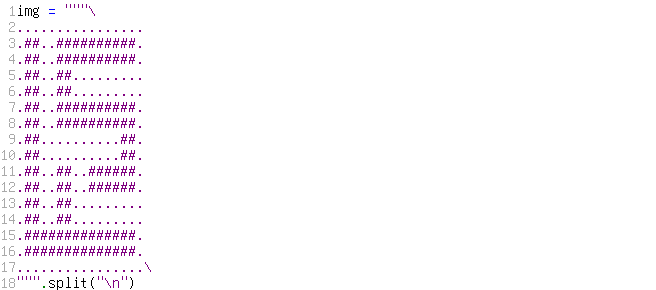Creative Commons

So, the moment we've all been waiting for, the ultimate equation of the Future Sketches logo is, as follows:

z = - 0.3 + 1.92 y^1 - 13.38 y^2 - 5.58 y^3 + 154.91 y^4 - 28.57 y^5 + 583.79 y^6 + 82.11 y^7 - 4144.24 y^8 - 7.35 x^1 + 0.03 x^1 y^1 + 408.01 x^1 y^2 - 6.02 x^1 y^3 - 6139.23 x^1 y^4 + 57.77 x^1 y^5 + 33906.89 x^1 y^6 - 137.15 x^1 y^7 - 61658.95 x^1 y^8 + 21.72 x^2 - 310.04 x^2 y^1 + 1878.12 x^2 y^2 + 9648.3 x^2 y^3 - 42106.62 x^2 y^4 - 81340.43 x^2 y^5 + 248029.92 x^2 y^6 + 191154.32 x^2 y^7 - 444357.34 x^2 y^8 + 164.59 x^3 - 9.16 x^3 y^1 - 9372.08 x^3 y^2 + 1655.07 x^3 y^3 + 138458.44 x^3 y^4 - 15870.74 x^3 y^5 - 751446.12 x^3 y^6 + 37676.8 x^3 y^7 + 1348650.85 x^3 y^8 - 576.95 x^4 + 2910.52 x^4 y^1 - 30087.87 x^4 y^2 - 126958.79 x^4 y^3 + 684990.41 x^4 y^4 + 1121251.81 x^4 y^5 - 4168580.6 x^4 y^6 - 2645084.55 x^4 y^7 + 7790980.56 x^4 y^8 - 1116.36 x^5 + 178.94 x^5 y^1 + 66836.01 x^5 y^2 - 32347.65 x^5 y^3 - 952729.57 x^5 y^4 + 310186.69 x^5 y^5 + 4987776.16 x^5 y^6 - 736376.68 x^5 y^7 - 8701328.23 x^5 y^8 + 5113.14 x^6 - 6815.04 x^6 y^1 + 157550.1 x^6 y^2 + 575691.26 x^6 y^3 - 3733812.39 x^6 y^4 - 5362274.5 x^6 y^5 + 23337034.87 x^6 y^6 + 12702399.32 x^6 y^7 - 45015333.72 x^6 y^8 + 2305.5 x^7 - 572.3 x^7 y^1 - 143703.48 x^7 y^2 + 103457.29 x^7 y^3 + 1991231.75 x^7 y^4 - 992068.29 x^7 y^5 - 10111735.53 x^7 y^6 + 2355149.29 x^7 y^7 + 17196162.38 x^7 y^8 - 12803.7 x^8 - 17.32 x^8 y^1 - 265602.05 x^8 y^2 - 886733.45 x^8 y^3 + 6631841.3 x^8 y^4 + 8717422.38 x^8 y^5 - 42682133.81 x^8 y^6 - 20732302.69 x^8 y^7 + 84921971.44 x^8 y^8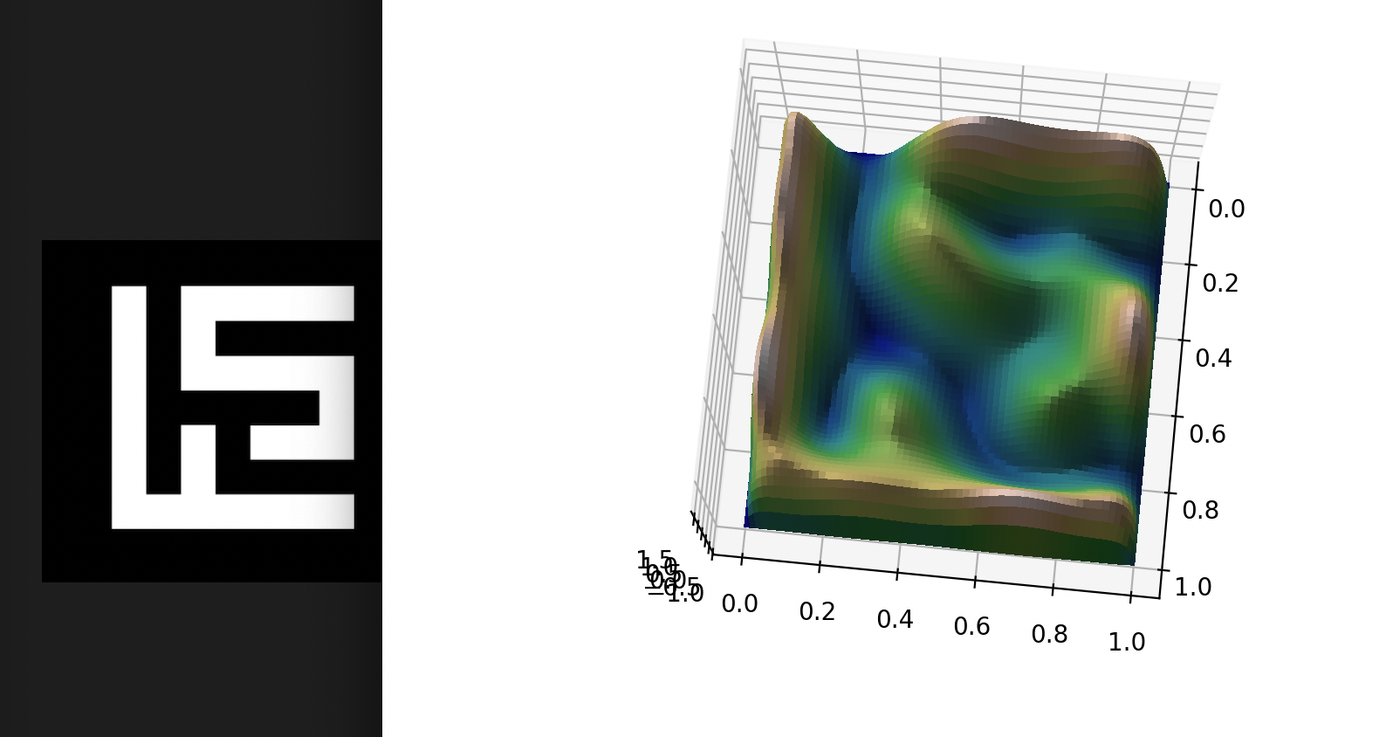Creative Commons

With these successful experiments in python, next I worked to port the algorithm to openFrameworks, where I can conduct even more interesting experiments, with shaders!

Eigen is a C++ linear algebra library that I heard a lot of good things about. It's also header only, so it should be quite convenient to include into an openFrameworks project. Therefore I decided to give it a try. I followed the examples  on their documentation for least square approximation. The SVD method doesn't quite compile (perhaps a typo in the example?) but the QR method worked well. So that's cool.

Given "data", a flattened array of pixel values 0/1 of dimension W * W, this code finds a matrix "m"  containing the coefficients for the polynomial surface.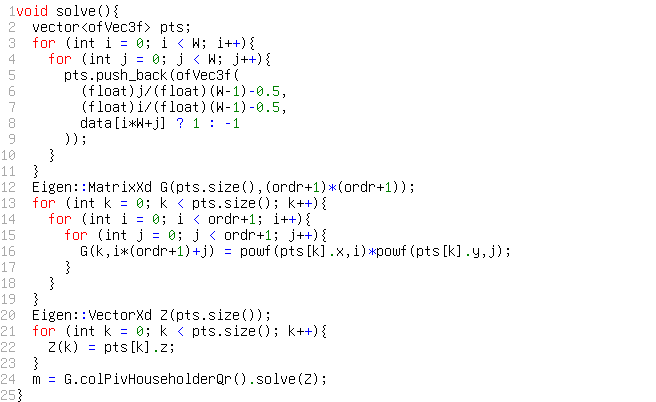Creative Commons

Next, I wrote a shader to display the surface given all 81 coefficients. (Yeah, it's pretty crazy).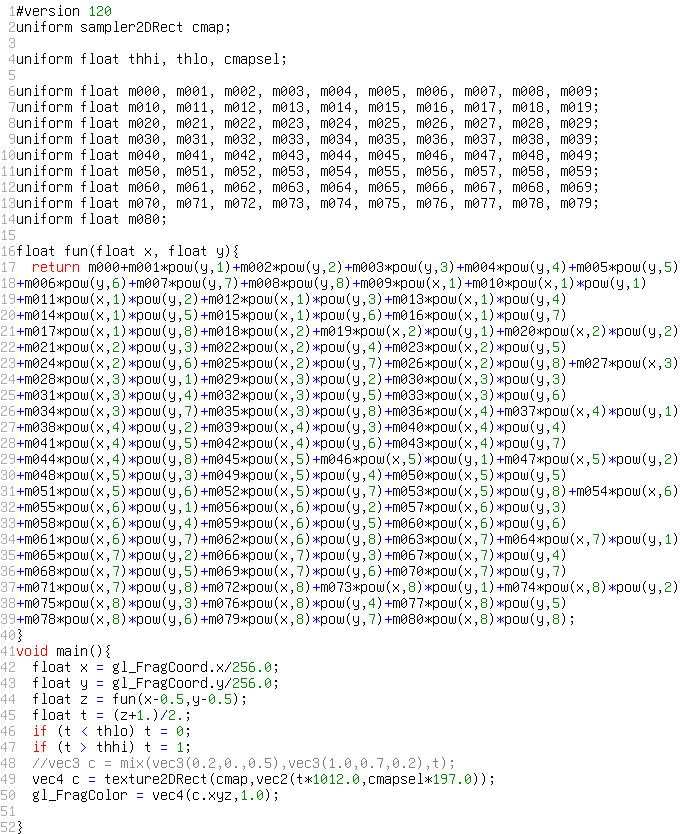Creative Commons

With the math out of the way, now we can have some fun. For instance, don't forget that we can fit a polynomial to any arbitrary binary image, and not just the Future Sketches logo! So I added an interface that allows you to paint anything with your mouse on a 7x7 grid, to which my program fit a surface, in real time.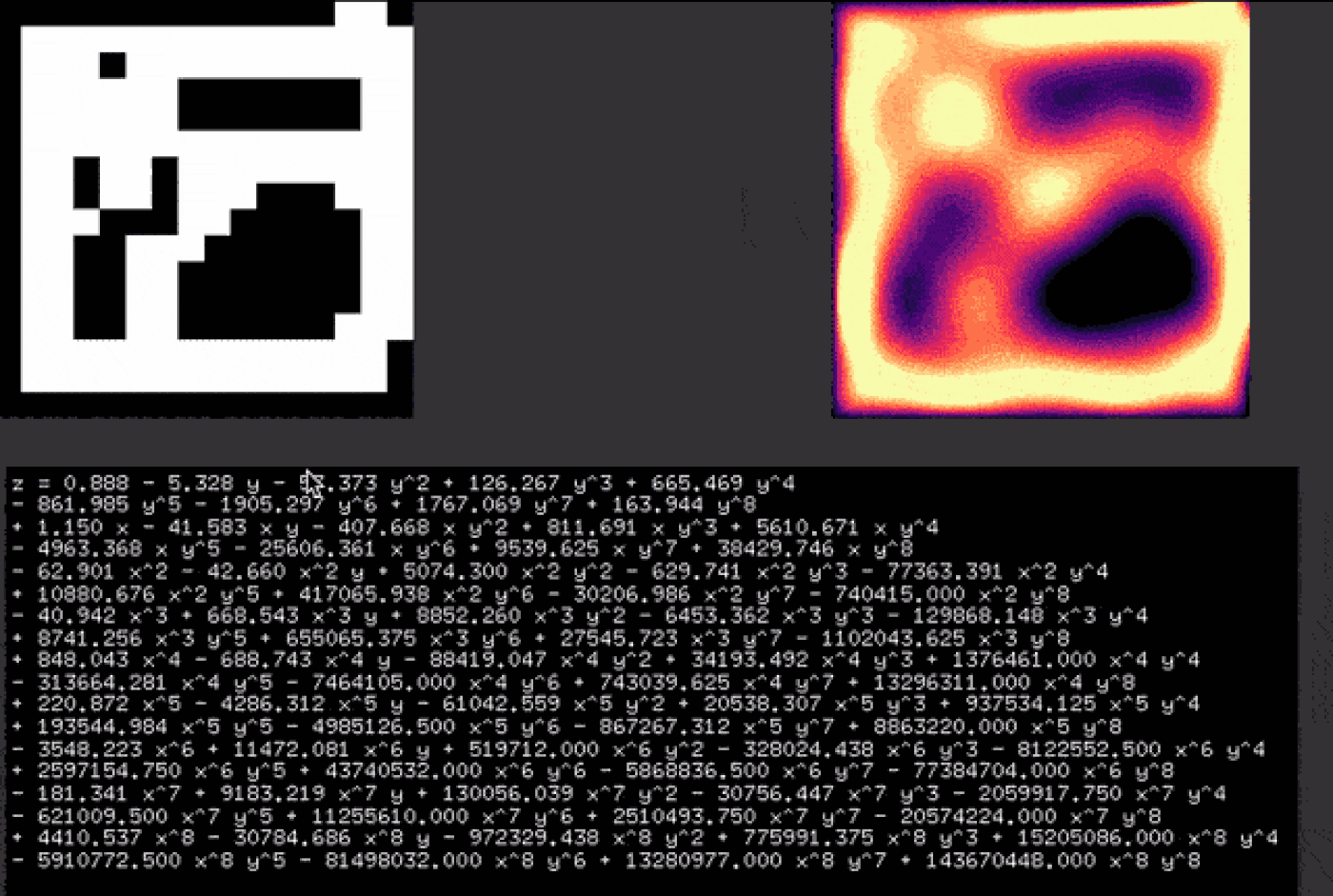Creative Commons

Moreover, we inadvertently discovered a new method to interpolate between images: instead of interpolating the value of each pixel, we can interpolate the coefficient of the polynomials!

I computed the polynomial for each of the 21 Media Lab group logos + the Media Lab logo, and you can interpolate between any two of them through interpolating polynomial coefficients.Creative Commons

It reminds me of GAN's, where one can walk in the latent space; Here, we can walk in the polynomial coefficient space.

View more